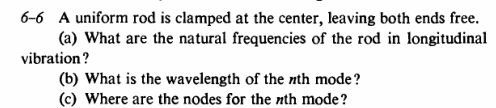# Waves on a metal rod

LCSphysicist
Homework Statement:
I will post an image right below.
Relevant Equations:
All below.I am trying to solve this question by ξ = A*cos(ωt + θ)*sin(kx + Φ)
Anyway, the two initial terms of the product helps nothing (i think), what matters is sin(kx + Φ)
So, i tried by two ways:

First:

The stress is essentially zero on the ends, that is, something like cte*∂ξ/∂x (strain) would be zero, so
L is the length of the rod.

cos(kx + Φ) need to be 0
Φ = (2n-1)*π
kL + Φ = (2n-1)*π

It is not good.

Second:

The half length position would carry a node, so
ξ = A*cos(ωt + θ)*sin(kL/2 + Φ) = 0
KL/2 + Φ = (n)*π
and with this i can not solve without Φ in the expression.

Homework Helper
Gold Member
2022 Award
I assume ξ is displacement. You would find it easier with x measured from the centre of the rod.

•LCSphysicist
LCSphysicist
I assume ξ is displacement. You would find it easier with x measured from the centre of the rod.
Definitively is easier deal with x measured from the center of the rod, but what is wrong in the above approach?